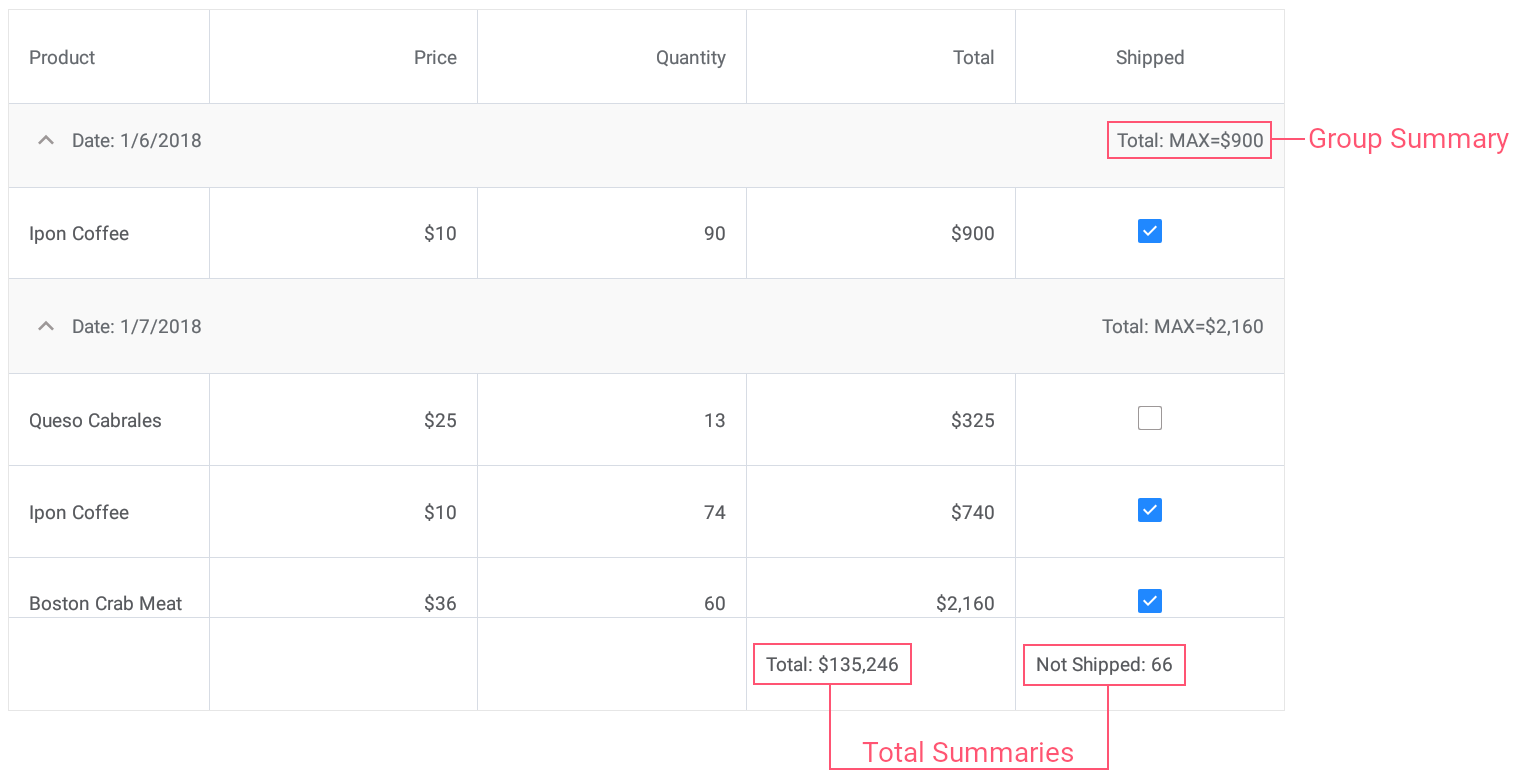21.1
20.2
20.1
19.2
19.1
1.1
The page you are viewing does not exist in version 1.1. This link will take you to the root page.

# CustomSummaryEventArgs.TotalValue Property

Gets or sets the summary value.

Namespace: DevExpress.XamarinForms.DataGrid

Assembly: DevExpress.XamarinForms.Grid.dll

## Declaration

``public object TotalValue { get; set; }``

## Property Value

Type Description
Object

An object that specifies the summary value.

## Remarks

TotalValue is the summary value that accumulates the custom calculated value. Note that this property should be initialized before the summary calculation process starts. To identify the status of the custom summary calculation, use the CustomSummaryEventArgs.SummaryProcess property. When performing calculations, you need to refresh the TotalValue property for it to be updated with the new values.

You can also modify the TotalValue property’s value when finalizing the calculation. In this case, the SummaryProcess property returns the Finalize value.

## Example

This example demonstrates how to use predefined aggregate functions and custom rule to calculate group and total summaries for grid columns.• Set the group summary to display the maximum Total value for each group of records.
• Set the total summary to calculate the sum of values in the Total column.
• Set the custom total summary to count the number of orders whose value in the Shipped column is false (to count orders that are not shipped).
``````<dxg:DataGridView x:Name="grid" ItemsSource="{Binding Orders}"
CalculateCustomSummary="OnCalculateCustomSummary">
<!-- ... -->
<dxg:DataGridView.GroupSummaries>
<dxg:GridColumnSummary FieldName="Total" Type="Max"/>
</dxg:DataGridView.GroupSummaries>

<dxg:DataGridView.TotalSummaries>
<dxg:GridColumnSummary FieldName="Total" Type="Sum"
DisplayFormat="Total: {0:C0}"/>
<dxg:GridColumnSummary FieldName="Shipped" Type="Custom"
DisplayFormat="Not Shipped: {0}"/>
</dxg:DataGridView.TotalSummaries>
</dxg:DataGridView>
``````
``````int count;
// ...

void OnCalculateCustomSummary(object sender, CustomSummaryEventArgs e) {
if (e.FieldName.ToString () == "Shipped")
if (e.IsTotalSummary){
if (e.SummaryProcess == CustomSummaryProcess.Start) {
count = 0;
}
if (e.SummaryProcess == CustomSummaryProcess.Calculate) {
if (!(bool)e.FieldValue)
count++;
e.TotalValue = count;
}
}
}
``````
See Also